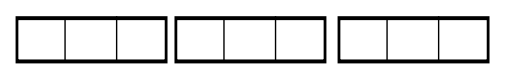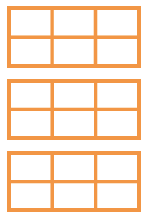### Home > CC1MN > Chapter 9 > Lesson 9.2.1 > Problem9-44

9-44.

Draw rectangles to represent each integer, then divide them into the fractional parts indicated in the problem. Part (a) is done as an example.

1. How many one-thirds are in $3$? That is, what is $3\div\frac{1}{3}$?As you can see in the figure above, each rectangle represents $1$, and each $1$ is divided into three parts.
If you count the thirds represented, you will find the answer to part (a).

2. How many one-fourths are in $5$? That is, what is $5\div\frac{1}{4}$?

Try drawing a diagram as modeled in part (a).
It may help to know that there are $4$ one-fourths in $1$.
Can you find how many are in $5$?

There are $20$ fourths in $5$.

3. How many one-sixths are in $3$? That is, what is $3\div\frac{1}{6}$?

Can you count the one-sixths in the three rectangles?4. How many one-fifths are in $6$? That is, what is $6\div\frac{1}{5}$?

The quotient is $30$.